### Seeing is believing!

Before you order, simply sign up for a free user account and in seconds you'll be experiencing the best in CFA exam preparation.

##### Subject 4. Measures of Center Tendency
Measures of central tendency specify where data are centered. They attempt to use a typical value to represent all the observations in the data set.

Population Mean

The population mean is the average for a finite population. It is unique; a given population has only one mean.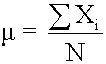where:

• N = the number of observations in the entire population
• Xi = the ith observation
• ΣXi = add up Xi, where i is from 0 to N

Sample Mean

The sample mean is the average for a sample. It is a statistic and is used to estimate the population mean.where n = the number of observations in the sample

Arithmetic Mean

The arithmetic mean is what is commonly called the average. The population mean and sample mean are both examples of the arithmetic mean.

• If the data set encompasses an entire population, the arithmetic mean is called a population mean.
• If the data set includes a sample of values taken from a population, the arithmetic mean is called a sample mean.

This is the most widely used measure of central tendency. When the word "mean" is used without a modifier, it can be assumed to refer to the arithmetic mean. The mean is the sum of all scores divided by the number of scores. It is used to measure the prospective (expected future) performance (return) of an investment over a number of periods.

• All interval and ratio data sets (e.g., incomes, ages, rates of return) have an arithmetic mean.
• All data values are considered and included in the arithmetic mean computation.
• A data set has only one arithmetic mean. This indicates that the mean is unique.
• The arithmetic mean is the only measure of central tendency where the sum of the deviations of each value from the mean is always zero. Deviation from the arithmetic mean is the distance between the mean and an observation in the data set.

The arithmetic mean has the following disadvantages:

• The mean can be affected by extremes, that is, unusually large or small values.
• The mean cannot be determined for an open-ended data set (i.e., n is unknown).

Geometric Mean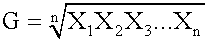The geometric mean has three important properties:

• It exists only if all the observations are greater than or equal to zero. In other words, it cannot be determined if any value of the data set is zero or negative.
• If values in the data set are all equal, both the arithmetic and geometric means will be equal to that value.
• It is always less than the arithmetic mean if values in the data set are not equal.

It is typically used when calculating returns over multiple periods. It is a better measure of the compound growth rate of an investment. When returns are variable by period, the geometric mean will always be less than the arithmetic mean. The more dispersed the rates of returns, the greater the difference between the two. This measurement is not as highly influenced by extreme values as the arithmetic mean.

Weighted Mean

The weighted mean is computed by weighting each observed value according to its importance. In contrast, the arithmetic mean assigns equal weight to each value. Notice that the return of a portfolio is the weighted mean of the returns of individual assets in the portfolio. The assets are weighted on their market values relative to the market value of the portfolio. When we take a weighted average of forward-looking data, the weighted mean is called expected value.

Example

A year ago, a certain share had a price of \$6. Six months ago, the same share had a price of \$6.20. The share is now trading at \$7.50. Because the most recent price is the most reliable, we decide to attach more relevance to this value. So, suppose we decide to "weight" the prices in the ratio 1:2:4, so that the current share price is twice as important as the price from six months ago, which in turn is twice as important as the price from last year.

The weighted mean would then be: (1 x 6 + 2 x 6.2 + 4 x 7.5) / (1 + 2 + 4) = \$6.91. If we calculated the mean without weights, we'd get: (6 + 6.2 + 7.5) / 3 = \$6.57. The fact that we've given more importance to the most recent (higher) share price inflates the weighted mean relative to the un-weighted mean.

Median

In English, the word "mediate" means to go between or to stand in the middle of two groups, in order to act as a referee, so to speak. The median does the same thing; it is the value that stands in the middle of the data set, and divides it into two equal halves, with an equal number of data values in each half.

To determine the median, arrange the data from highest to lowest (or lowest to highest) and find the middle observation. If there are an odd number of observations in the data set, the median is the middle observation (n + 1)/2 of the data set. If the number of observations is even, there is no single middle observation (there are two, actually). To find the median, take the arithmetic mean of the two middle observations.

The median is less sensitive to extreme scores than the mean. This makes it a better measure than the mean for highly skewed distributions. Looking at median income is usually more informative than looking at mean income, for example. The sum of the absolute deviations of each number from the median is lower than the sum of absolute deviations from any other number.

Note that whenever you calculate a median, it is imperative that you place the data in order first. It does not matter whether you order the data from smallest to largest or from largest to smallest, but it does matter that you order the data.

Mode

Mode means fashion. The mode is the "most fashionable" number in a data set; it is the most frequently occurring score in a distribution and is used as a measure of central tendency. A set of data can have more than one mode, or even no mode. When all values are different, the data set has no mode. When a distribution has one value that appears most frequently, it is said to be unimodal. A data set that has two modes is said to be bimodal.

The advantage of the mode as a measure of central tendency is that its meaning is obvious. Like the median, the mode is not affected by extreme values. Further, it is the only measure of central tendency that can be used with nominal data. The mode is greatly subject to sample fluctuations and, therefore, is not recommended for use as the only measure of central tendency. A further disadvantage of the mode is that many distributions have more than one mode. These distributions are called "multimodal."

Harmonic Mean

The harmonic mean of n numbers xi (where i = 1, 2, ..., n) is: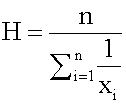The special cases of n = 2 and n = 3 are given by: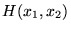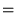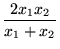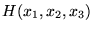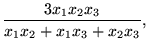and so on.

For n = 2, the harmonic mean is related to arithmetic mean A and geometric mean G by: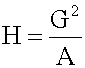The mean, median, and mode are equal in symmetric distributions. The mean is higher than the median in positively skewed distributions and lower than the median in negatively skewed distributions. Extreme values affect the value of the mean, while the median is less affected by outliers. Mode helps to identify shape and skewness of distribution.

Learning Outcome Statements

e. calculate and interpret measures of central tendency, including the population mean, sample mean, arithmetic mean, weighted average or mean, geometric mean, harmonic mean, median, and mode;

m. compare the use of arithmetic mean and geometric means when analyzing investment returns.

CFA® 2021 Level I Curriculum, , Volume 1, Reading 7

User Comment
MURF how do you do a geometric mean with a negative number?? 5, -3, 6
chandos it cannot be calculated if one of the values is -ve
aggieguy05 it can too be done. (1.05*.97*1.06)=g^3
db28luke you add 1 to each return and take the nth root minus 1
db28luke its in the book...page 125
mogulcn in the case above, it is still positive as the data set are 1+Rt. in the book, it is said that the observations will never be negative becasue the biggest negative return is -100%
achu think of geometric mean as something like "multiplicative mean" average- product of n items then taken to 1/n th power.
valerycfa When calculating variance, why do we loose a degree of freedom when passing from population to sample calculation ?
Mariecfa If the sample variance were defined with division by n, it would systematically underestimate the value of the population variance. So, we compensate by increasing its overall value by making its denominator smaller (by using n-1 instead of n). Division by (n-1) causes the sample variance to target the value of the population variance, whereas division by (n) causes the sample variance to underestimate the value of the population variance.
AmyJ How do you solve for Geometric mean with an HP 12C calculator? Thank you.
boddunah amyJ

hp 12c platinum solution for geometric return.

for example yearly returns are 5%,(3%),2%
geometric return as follows
step 1 :1.05*0.97*1.02 = 1.038870. (3% is negative return. so 1-0.03=.97)
step 2: enter 3 and press button 1/x .result = 0.3333.(we used 3 bc 3 years returns were given)
step 3 :0.3333 (already entered) press button Y^x. It should give you 1.0128.
step 4 : subtract 1 from 1.0128 = 0.0128 0r 1.28% geometric return.
jpducros What do we use an harmonic mean for ?
knowles242 as jpducros indicated is there an application of the harmonic mean?
Mosobalaje Harmonic mean is generally used to measure average investment costs over a time period. It's not used to calculate returns.
Barchie Why is it that the geometric mean is not as affected by the extremes? (that is it's advantage, I just don't get why not.)
birdperson 2 other "fun" facts
-- sum of deviations from the (arithmetic) mean = 0
-- when the values are positive and not equal, H < G < A
Kholofelo Another way to look at the typical exam question is to say

If this were a normal distribution my average would be 100

Using 80 as a spread either way (20 + 180) / 2 = 100
OR
using 100 as a spread either way (0 + 100) / 2 = 100

By having 20 has my lower limit and 200 as my upper limit, my average is pulled "upward" or to the right of the mean i.e. (20 + 200) / 2 = 110 hence it is skewed to the right of the mean.
Kholofelo *(0 + 200) / 2 = 100
unknown the geometric mean of 5 variables can be done (x1*x2*x3*x4*x5)^1/5,
because the square root in BAII is standardized for one underlying.